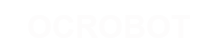#### Site Tools

en:reference:language:boolean

# Differences

This shows you the differences between two versions of the page.

 — en:reference:language:boolean [2017/04/07 10:03] (current) 2014/11/13 14:39 弘毅 created 2014/11/13 14:39 弘毅 created Line 1: Line 1: + ====== Boolean Operators ====== + + + These can be used inside the condition of an if statement. + ===== && (logical and) ===== + + True only if both operands are true, e.g. + <​code>​ + if (digitalRead(2) == HIGH  && digitalRead(3) == HIGH) { // read two switches ​ + // ... + } + ​ + is true only if both inputs are high. + ===== || (logical or) ===== + + True if either operand is true, e.g. + <​code>​ + if (x > 0 || y > 0) { + // ... + } + ​ + is true if either x or y is greater than 0. + ===== ! (not) ===== + + True if the operand is false, e.g. + <​code>​ + if (!x) { + // ... + } + ​ + is true if x is false (i.e. if x equals 0). + ===== Warning ===== + + Make sure you don't mistake the boolean AND operator, && (double ampersand) for the bitwise AND operator & (single ampersand). They are entirely different beasts. + + + Similarly, do not confuse the boolean || (double pipe) operator with the bitwise OR operator | (single pipe). + + + The bitwise not ~ (tilde) looks much different than the boolean not ! (exclamation point or "​bang"​ as the programmers say) but you still have to be sure which one you want where. + ===== Examples ===== + <​code>​ + if (a >= 10 && a <= 20){}   // true if a is between 10 and 20 + ​ +